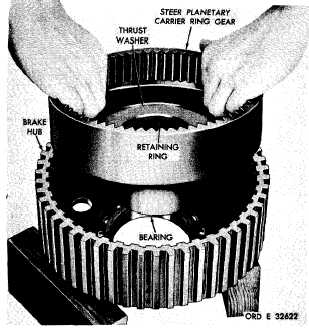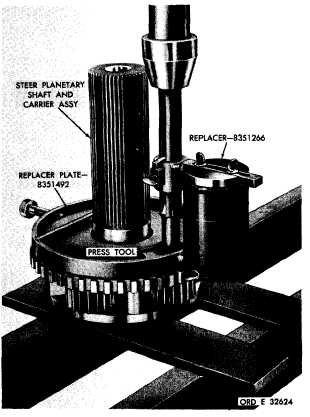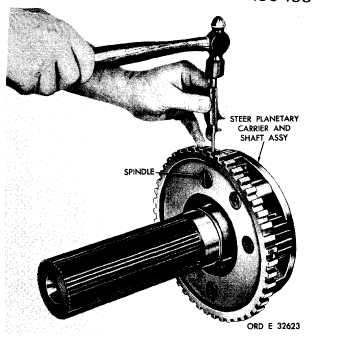Custom SearchC H A P    5,     S E C     X I X L E F T ,         R I G H T     S T E E R     P L A N E T A R I E S     R E B U I L D PAR  8 - 1 60 Figure   203.   Removing   (or   installing)   steer   planetary carrier ring gear assembly .   U s i n g    a    h a m m e r    a n d    p u n c h ,    r e m o ve four   steer   planetary   carrier   spindle   lock   pins (fig.    204). h  .   U s i n g     r e p l a c e r     p l a t e     8 3 5 1 4 9 2 ,     r e- placer   8351266   and   a   suitable   press   tool,   press four   spindles   from   the   planetary   shaft   and   car- r i e r    a s s e m b l y    ( f i g .    2 0 5 ). .   R e m o v e    t h e    s t e e r    p l a n e t a r y    c a r r i e r pinions   (6,   fig.   378,   fold-out   7),   roller   bear- ings   7,   spacers   5   and   8,   and   thrust   washers 4   and   9   from   carrier   3. 1 5 9 .     C L E A N I NG Refer   to   par.   71   for   cleaning   recommen- d a t i o n s . 1 6 0 .    I N S P E C T I O N    A N D    R E P A IR R e f e r    t o    p a r .    7 2    f o r    g e n e r a l    i n s p e c t i o n and    repair    recommendations.    Repair    and    re- b u i l d    p o i n t s    o f    m e a s u r e m e n t    f o r    f i t s ,    c l e a r- ances   and   wear   limits   are   indicated   by   small, lower   case   letters   in   figs.   378   and   380,   fold- outs   7   and   9.   Refer   to   pars.   241   and   243   for wear    limits    information. 1 4 0 Figure  204.  Removing  (or  installing)  spindle  lock  pin Figure  205.  Removing  steer  planetary  carrier  and  shaft assembly  spindle